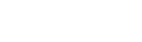# Highlight Part of a Map based on Drop Down Selection

Here is a neat trick for people who use maps in Excel. In this blog, I will explain how to highlight map in excel based on a drop down selection.

I have taken an editable map of the US. The idea is to highlight a state in the US when its name is selected from a drop down.

Something as shown in the pic below:Now before I show you the code, here are a few prerequisites for this trick.

1. Get an editable map of the US in which you can select different shapes that you want to highlight
2. Create a list of all the 50 states in one column, and on the column to its right I wrote State 1, State 2, and so on3. Name each shape on the map. For example, I have 50 shapes here for 50 states, and I have named each Shape as State 1, State 2, State 3, and so on..To do this, select any shape, and go to the Name Box, which is on the left of formula bar, and enter its name from the State Number column. For example, I selected Alabama and named it State 1.4. Create an Excel drop down list with the names of all the states (\$B\$2 in this case)5. Use the Vlookup function to extract the state number when a state is selected from the drop down. Here is the formula that I have used in cell \$B\$3
=VLOOKUP(B2,’State List’!\$B\$3:\$C\$52,2,FALSE)
##### Code to Highlight Map in Excel
```Private Sub Worksheet_Change(ByVal Target As Range)
Dim N As Integer
Dim ShapeName As String
N = ActiveSheet.Shapes.count
For i = 1 To N
ShapeName = ActiveSheet.Shapes(i).Name
If Left(ShapeName, 6) = "State " Then
ActiveSheet.Shapes(i).Select
With Selection.ShapeRange.Fill
.Visible = msoFalse
.Transparency = 1
End With
End If
Next i
StateNumber = Range("\$B\$3").Value
ActiveSheet.Shapes(StateNumber).Select
With Selection.ShapeRange.Fill
.Visible = msoTrue
.Visible = msoTrue
.ForeColor.RGB = RGB(192, 0, 0)
.Transparency = 0
.Solid
End With
ActiveSheet.Range("\$B\$2").Select
End If
End Sub```

Follow these simple steps while pasting this code

1. First, right-click on the sheet tab that has the map and select “View Code”. This will open the VB editor.2. Paste the code.FREE EXCEL BOOK

## Get 51 Excel Tips Ebook to skyrocket your productivity and get work done faster

### 5 thoughts on “Highlight Part of a Map based on Drop Down Selection”

1. Hi
In the code, Is there suppose to a space between “StateNumber” or…